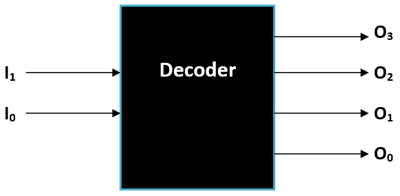# Difference between Encoder and Decoder

The encoder gives the binary codes in the form of output, whereas the decoder takes these binary codes. Both look similar, but there are several differences between encoder and decoder. You can read this article if you also want to know the significant difference between encoder and decoder. As here, we will not also discuss the critical differences but also understand about encoder and decoder too.

### What is Encoder?Encoding is converting text into the code format where the text is translated into binary form. The encoder can be explained as the mechanism that processes the encoding activity in the device and produces output as the binary code. In short, the encoder is the component or the hardware that can transform the digital signals into the coded format. The devices can also transform the analog signals into the coded variant of that. This process is done by encoder because the computer can not understand the digital or analog signals.

If the person needs to communicate through the devices, then that device needs to have an encoder. So the user can type their messages such as text messages, common search queries, or other terms. And the encoder can translate the text format into binary or coded form. However, the encoder is insufficient to complete the communication as this process also needs to be decoded.

### What is Decoder?Decoding is the process where the coded term translates again in the text format. And the decoder is the mechanism that executes this process accurately. It is also a combinational circuit used these days, and it works opposite to the encoder. It translates the coded forms into the n lines as the output and that users can easily understand. With this process, the communication gets completed from both sides, i.e., sender and receiver. If you receive the message in the text format and understand it, then it means the device you are using has the decoder.

The decoding process can be done either in the form of text format or in verbal format. Generally, the decoders use microprocessors and memory cards to complete this process.

## Difference between Encoder and Decoder

There are many significant differences between encoder and decoder, and here we will take a short glimpse of it. Thus, before we start discussing the comparison chart of encoder vs decoder.

• The encoder device converts the digital or analog signals into the code digit form. In contrast, the decoder is the device that interprets back from coded digits into original digital or analog signals.
• The encoder generally accepts the n power of 2 for encoding the message or information. But on the other hand, the decoder only uses the n number of units for decoding the codes.
• In most cases, the encoders are installed at the transmitting section of the communication system. But the decoder exists on the receiving side of the message or the hardware.
• The encoder mainly uses the n number of lines for the output, whereas the decoder uses the n power of 2.
• The encoder receives either digital or analog signals for the encoding message or information. The decoder receives the coded format to translate into the original signals.
• The encoding process is more straightforward when it comes to complexities, but translating coded digital into digital or analog signals is a challenging task. Hence the decoder has to conduct a more challenging process compared to the encoder.
• Along with all of these, encoding is recognized as the production of messages or information. In contrast, decoding is described as the interpretation of the coded messages.
 Basis Encoder Decoder Explanation The encoder is the device or the circuits that transform the digital or analog signals into the code format. The decoder is considered the combination of a decoder that decodes the coded digits into the original information as encoded. Used Logic In encoding, the OR gate is used as the base-level logic element to transform the information into the code. AND gate and NOT gate are the commonly used and base level decoding logic in most of the decoding process. Input Line The encoder accepts the 2n number of input to process in the form of binary code. But the decoder only accepts the n number of lines for the decoding. Input Information Here the digital or analog signals come into use as the input of the encoder. In decoding, the coded binary becomes the input of the decoder. Operational Activities The operation of the encoder is simple compared to the decoder. The activity decoded by the decoder is a bit complex compared to encoding. As it requires a sound understanding of coding and code programs. Installation Process The encoder is generally found at the end of the transmission end in the communication system. The decoder is generally placed at the receiving side of the communication system. Application Used The encoding applications are email, audio encoders, video encoders, and more. Here microprocessors, memory chips are significant examples of decoding applications. Output Line For the output line, the encoder mainly presents the n number of lines. For the output, the decoder shows 2n numbers of lines of the information.

### Conclusion

So, the encoder is the device that is used to translate the standard text into binary codes. That can also be explained as the encoder transforming the digital or analog information into the coded format. On the other hand, the decoder is the device used to translate the code again in the format of the original information. The encoder is the device that processes the coded digital to translate into the original signals so the user can understand.

We learned about the difference between encoder and decoder using a comparison table. Moreover, we also covered several other foundational terminologies that you are familiar with now. Thus, if you love this exciting information about encoder vs decoder, then show your knowledge in the comment section.